## Saturday, August 31, 2019

I've been fascinated by Hadamard Transforms since I first ran into them as an undergraduate. Think of them as like Fourier transforms where the basis functions are rectangular waves (+1/-1) rather than sinusoids (complex exponentials) as in the Fourier. But there are strong parallels: both use orthonormal basis functions and there's even a Fast Hadamard Transform that is exactly analogous to the FFT. Back in the day of limited hardware, there was considerably more interest in the Hadamard transform as it could be computed mostly with additions rather than the vastly more expensive multiplication by transcendentals.

Here is the Hadamard basis functions for a 64 x 64 two-dimensional transform. It's not obvious, but the rows and columns are "orthogonal" meaning if you multiply and sum any two columns or rows, the result will be zero. (Note that black elements are -1, not zero, though white elements are +1.)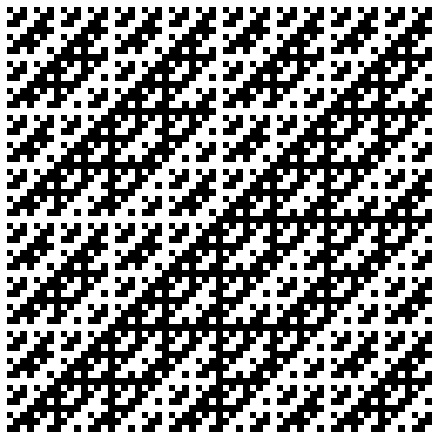It's more useful to consider the bases in "sequency" ordering -- with analogy to frequency, the sequency increases from left to right and top to bottom. The highest sequency is a square wave, while the lowest is DC -- a constant. Though intermediate sequency bases look much like square waves, most of them are not because of the orthogonality requirement.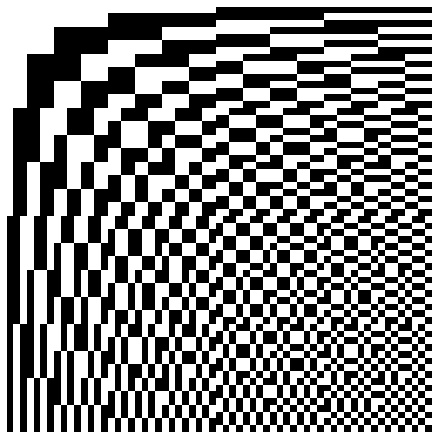Compare withe the Fourier bases from here. Since these are complex they've been decomposed into even (cosine) and odd (sine) components so they may be imaged.With nearly perfect analogy to the Discrete Cosine Transform, the Hadamard transform can be used for compression and filtering, though in the "sequency* domain as opposed to the frequency domain. Let's look at compression first. Starting with this 512 x512 image:If we take the Hadamard transform and retain just the highest-magnitude coefficients, we get these images, corresponding to .014%, 0.5%, and 5.2% compression. Not bad for just additions!Now we can look at filtering in the sequency domain. Here's an image of the cameraman image in the sequency-ordered Hadamard domain (this is hard to display; here I've imaged the log of the absolute values plus epsilon, and stretched the contrast).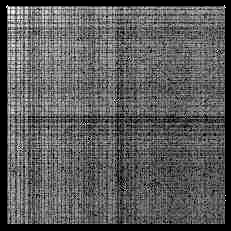If we zero out the low-sequency coefficients, we get this (here black is zero):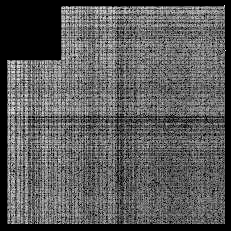And taking the inverse Hadamard transform (which, except for a scale factor, is the same as the forward), we get a high-pass filtered version with no low-sequency components: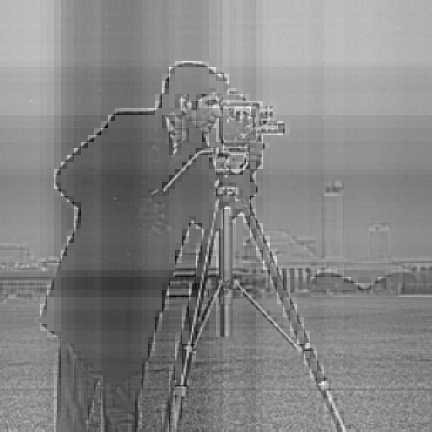Here are a couple other sequency-based filters, from left to right a lowpass in the horizontal direction, a lowpass in the vertical direction.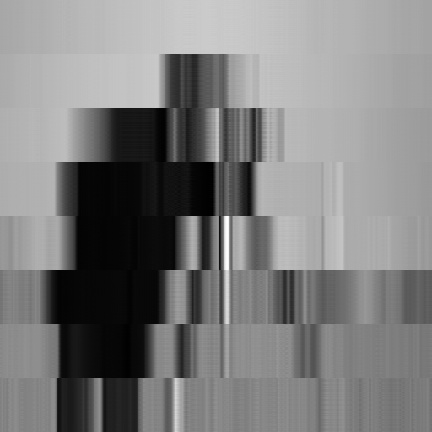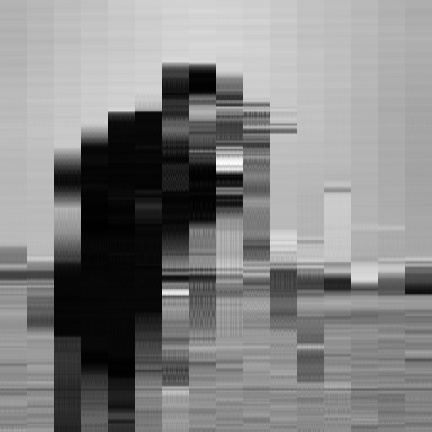And finally, an example of an image glitched in the sequency domain. Here, instead of zeroing out the low-sequency components, we've inverted their sign.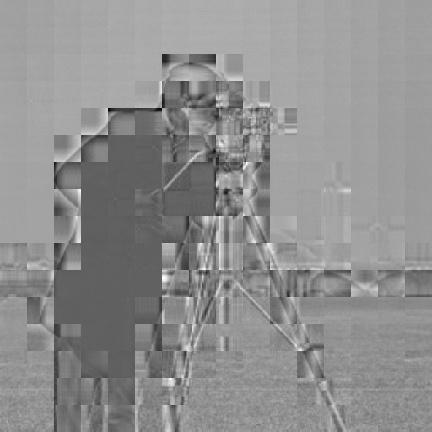Though it's a little messy, here is the Python code in a Jupyter notebook.

# Topics: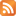RSS feed